# Texas Go Math Grade 3 Lesson 18.6 Answer Key Metric Units for Liquid Volume

Refer to our Texas Go Math Grade 3 Answer Key Pdf to score good marks in the exams. Test yourself by practicing the problems from Texas Go Math Grade 3 Lesson 18.6 Answer Key Metric Units for Liquid Volume.

## Texas Go Math Grade 3 Lesson 18.6 Answer Key Metric Units for Liquid Volume

Unlock the Problem

Liquid volume is the amount of liquid in a container. The liter (L) is the basic metric unit for measuring liquid volume. A milliliter (mL) is the metric unit to measure liquid in very small containers.

Activity

Materials; 1-L beaker; a bottle cap; 1-mL dropper; 2 containers; water; tape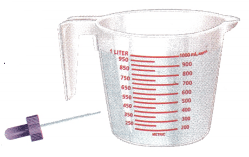STEP 1:
Fill a 1-liter beaker with water to the 1-liter mark.

STEP 2:
Pour 1 liter of water to fill a container. Mark the level of the water with a piece of tape. Draw the container below and name the container. Write if the container holds less than a liter, a liter, or more than a liter.

STEP 3:
Repeat Steps 1 and 2 with a different-sized container.
Container 1
Container 2

STEP 4:
Use the 1-mL dropper filled with water to fill the bottle cap. How many times did you fill the dropper?

Explain if the bottle cap is less than a milliliter, a milliliter, or more than a milliliter? ____________

Name a container that will hold a liter of water.
Will the container hold 50 bottle caps of water? Justify.
Container 1 is a cooking pot; more than a liter.
Container 2 is a vase of less than a liter.
A water bottle will hold a liter of water.
We can fill the dropper 20 times with 1-mL dropper filled with water to fill the bottle cap.

Explanation:
Yes. The container holds at least a liter of water, a bottle cap holds a few milliliters of water which is a very small part of a liter. So, the container will hold 50 bottle caps of water.

Math Talk
Mathematical Processes

What can you say about the amount of liquid volume in each container?Suppose you drank a glass of orange juice. Did you drink about 4 mL or 300 mL of orange juice? Explain.
The 4 ml would only be a few droppers full of juice and a glass would contain much more liquid, so 300 mL is a better to estimate.

Share and Show

Choose the unit you would use to measure each container’s capacity. Write milliliter or liter.

Question 1.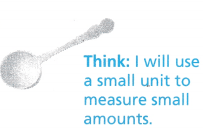milliliter

Question 2.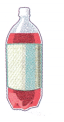liter

Question 3.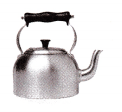liter

Question 4.liter

Question 5.milliliter

Question 6.liter

Ginger pours punch into three 1-L bottles. Choose the metric unit to measure the liquid volume in each bottle. Write milliliter or liter.

Question 7.milliliter

Question 8.liter

Question 9.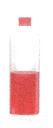milliliter

Problem Solving

Use the containers for 10-13. Container A is full when 1 liter of water is poured into it.Question 10.
Write Math What if you poured 1 liter of water into Container B? Describe the way the water fills the container. Explain how you know.
It will be almost full.

Explanation:
Container A holds 1 liter. Since Container B is shorter and wider, it will most likely hold the same amount of water.

Question 11.
H.O.T. Reasoning Bryson filled 2 containers full with water. One container held 2 liters and the other container held 250 milliliters. Which two containers did he fill?
Container C and Container E.

Explanation:
Container C would hold 2 liters of water. Container E would hold 250 milliliters of water. So we compared C and E to A.

Question 12.
H.O.T. Evaluate Name two containers that will be filled with about the same number of liters of water. Explain.
Containers A and B.

Explanation:
Container A is taller than Container B, but not as wide.

Question 13.
H.O.T. What’s the Error? Samuel says that you can pour more liters of water into Container B than into Container D. Is he correct? Explain.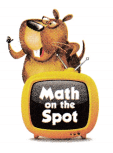No

Explanation:
Container B is smaller than container D. Therefore Container D is much taller, it can hold more liters of water when filled.

Fill in the bubble for the correct answer choice.

Question 14.
Representations For which container would you use milliliters to measure the amount of liquid?D

Question 15.
Which container has the greatest liquid volume when filled?
(A) pitcher
(B) mug
(C) bucket
(D) bottle cap
Bucket

Explanation:
The bucket container has the greatest liquid volume when filled.

Question 16.
Multi-Step If the liquid in two of the bottles are poured into another container, which two will have the least combined liquid volume?(A) C and D
(B) A and B
(C) A and C
(D) B and D
C and D

Explanation:
C and D will have the least combined liquid volume.

Texas Test Prep

Question 17.
The bottles of tea are all liter bottles. Which bottle has a liter of tea?(A) Bottle J
(B) Bottle K
(C) Bottle M
(D) Bottle L
Bottle M

Explanation:
Bottle M has a liter of Tea.

### Texas Go Math Grade 3 Lesson 18.6 Homework and Practice Answer Key

Choose the unit you would use to measure the capacity. Write milliliter or liter.

Question 1.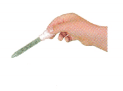milliliter

Question 2.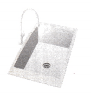liter

Question 3.liter

Question 4.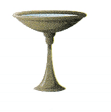liter

Question 5.milliliter

Question 6.milliliter

Problem Solving

Use the containers for 7-9. Container A is full when 1 liter of water is poured into it.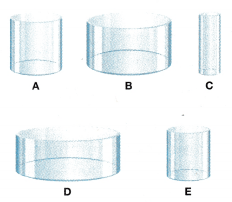Question 7.
What if you poured 1 liter of water into container C? Describe the way the water fills the container.
The water would flow all over the sides since container C is about the same height but thinner than container A

Question 8.
Which container would hold about half of the water as in container D? Explain.
Container E

Explanation:
Container E is about half as wide, but at the same height as container D.

Question 9.
For which containers would you use milliliters to measure the liquid volume? Explain.
Container C and E

Explanation:
C and E are smaller or thinner than A which holds one liter, so measure their liquid volume in milliliters.

Lesson Check

Question 10.
Which container has the least liquid volume when filled?
(A) water glass
(B) tablespoon
(C) soup bowl
(D) fish bowl
tablespoon

Explanation:
The Tablespoon container has the least liquid volume when filled.

Question 11.
Which container would you use a liter to measure the amount of liquid?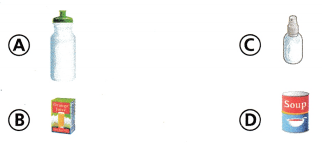A

Explanation:
I would use container Option A as a liter to measure the amount of liquid.

Question 12.
The bottles of liquid are all liter bottles. Which amount of liquid would fill a juice glass?Option B

Explanation:
Option B amount of liquid would fill a juice glass.

Question 13.
Multi-Step If the liquid in two of the containers is poured into another container, which two will have the greatest combined liquid volume?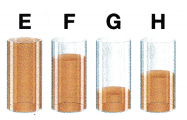(A) E and H
(B) F and H
(C) E and F
(D) G and H
E and F

Explanation:
E and F will have the greatest combined liquid volume.

Question 14.
Multi-Step Each of the containers has a capacity of 1 liter. If the liquid in two of the containers is poured into another container, which two will have a combined volume of 1 liter?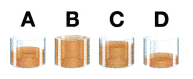(A) C and D
(B) B and C
(C) A and D
(D) B and D